# 1724 Carol of the Ukrainian Bells

### Today’s Puzzle:

As I sit in my warm, peaceful house, I often think about the people of Ukraine whose country has been ravaged by war. Many of them, including children, are also facing a winter with no heat. My heart goes out to them.

My childhood was so unlike theirs. I was able to attend school classes and learn about many different topics without fear of dying. In Junior High School choir class, one of the songs I learned was called Carol of the Bells. I just recently learned from Slate magazine of the song’s Ukrainian roots:

A little over a hundred years ago Mykola Leontovych, a Ukrainian composer, arranged several of his country’s folk songs together in a piece he titled Shchedryk. Tragically, he was murdered by a Russian assassin on January 23, 1921, in the Red Terror, when the Bolsheviks were intent on eliminating Ukrainian leaders, intellectuals, and clergy.

During this time of great unrest, the Ukrainian National Chorus performed Shchedryk around the world and in cities large and small in the United States. One performance was even given at the famed Carnegie Hall on October 5, 1922. The haunting melody was heard by Peter Wilhousky who penned alternate words for it: Hark how the bells, Sweet silver bells,…

Now it is one of our most beloved Christmas carols. I am grateful I learned the words and tune in junior high, although I wish I had learned of its Ukrainian history then as well.

These two bells puzzles are reminiscent of Ukraine’s flag. Long may it wave. Write each number 1 to 10 in the yellow columns and rows so that the given clues are the products of the numbers you write.Here are the same puzzles if you prefer to use less of your printer ink.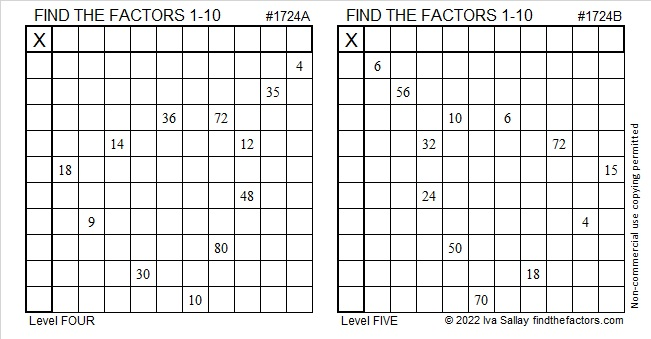### Factors of 1724:

• 1724 is a composite number.
• Prime factorization: 1724 = 2 × 2 × 431, which can be written 1724 = 2² × 431.
• 1724 has at least one exponent greater than 1 in its prime factorization so √1724 can be simplified. Taking the factor pair from the factor pair table below with the largest square number factor, we get √1724 = (√4)(√431) = 2√431.
• The exponents in the prime factorization are 2 and 1. Adding one to each exponent and multiplying we get (2 + 1)(1 + 1) = 3 × 2 = 6. Therefore 1724 has exactly 6 factors.
• The factors of 1724 are outlined with their factor pair partners in the graphic below.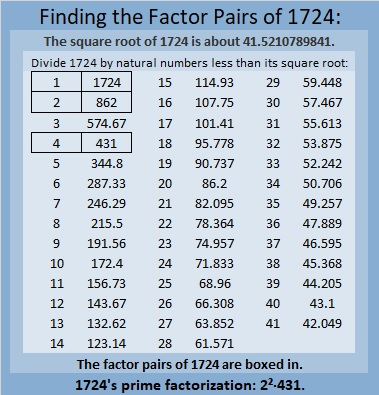### More About the Number 1724:

1724 is the difference of two squares:
432² – 430² = 1724.

1724₁₀ = 464₂₀, a palindrome, because
4(20²) + 6(20¹) + 4(20°) = 1724.

# 1704 Christmas Factor Tree

### Today’s Puzzle:

If you know the factors of the clues in this Christmas tree, and you use logic, it is possible to write each number from 1 to 12 in both the first column and the top row to make a multiplication table. It’s a level six puzzle, so it won’t be easy, even for adults, but can YOU do it?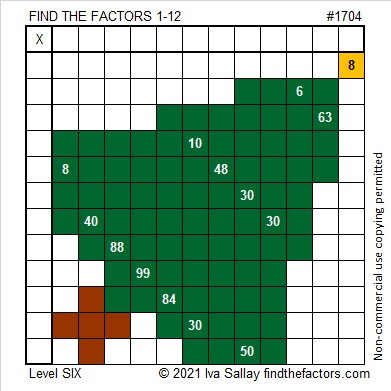### Factors of 1704:

If you were expecting to see a factor tree for the number 1704, here is one of several possibilities: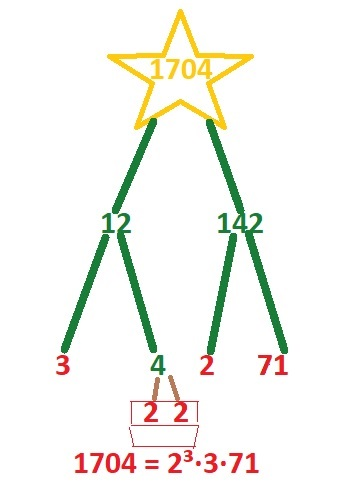• 1704 is a composite number.
• Prime factorization: 1704 = 2 × 2 × 2 × 3 × 71, which can be written 1704 = 2³ × 3 × 71.
• 1704 has at least one exponent greater than 1 in its prime factorization so √1704 can be simplified. Taking the factor pair from the factor pair table below with the largest square number factor, we get √1704 = (√4)(√426) = 2√426.
• The exponents in the prime factorization are 3,1 and 1. Adding one to each exponent and multiplying we get (3 + 1)(1 + 1) (1 + 1) = 4 × 2 × 2 = 16. Therefore 1704 has exactly 16 factors.
• The factors of 1704 are outlined with their factor pair partners in the graphic below.### More About the Number 1704:

1704 is the difference of two squares in FOUR different ways:
427² – 425² = 1704,
215² – 211² = 1704,
145² – 139² = 1704, and
77² – 65² = 1704.

Why was Six afraid of Seven? Because Seven ate Nine.
1704 is 789 in a different base:
1704₁₀ = 789₁₅ because 7(15²) + 8(15¹) + 9(15º) = 1704.

# 1568 A Challenging Christmas Tree

### Today’s Puzzle:

Can you write the numbers from 1 to 10 in each of the boldly outlined columns and rows so that each quadrant of this Christmas tree puzzle behaves like a multiplication table? Remember to use logic and not guessing and checking.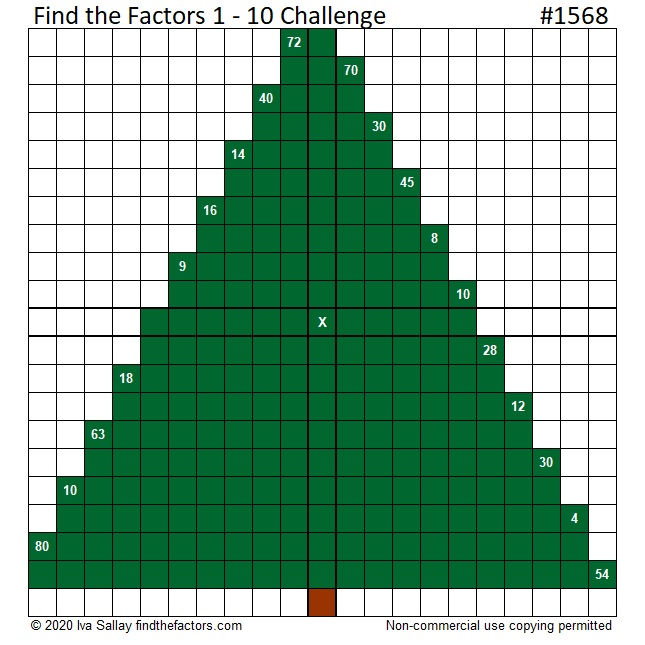Here is the same puzzle that won’t use so much ink to print:### 1568 Factor Tree:

Here is one of several possible factor trees for 1568: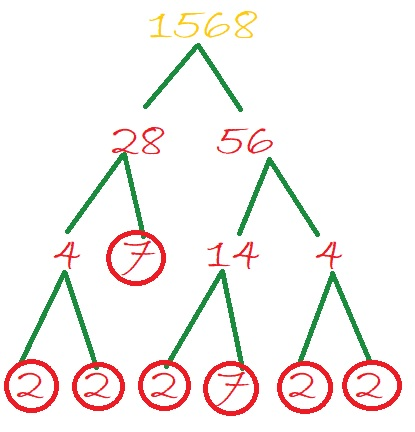### Factors of 1568:

• 1568 is a composite number.
• Prime factorization: 1568 = 2 × 2 × 2 × 2 × 2 × 7 × 7, which can be written 1568 = 2⁵ × 7².
• 1568 has at least one exponent greater than 1 in its prime factorization so √1568 can be simplified. Taking the factor pair from the factor pair table below with the largest square number factor, we get √1568 = (√784)(√2) = 28√2.
• The exponents in the prime factorization are 5 and 2. Adding one to each exponent and multiplying we get (5 + 1)(2 + 1) = 6 × 3 = 18. Therefore 1568 has exactly 18 factors.
• The factors of 1568 are outlined with their factor pair partners in the graphic below.### More about the Number 1568:

1568 is the difference of two squares SIX different ways:
393² – 391² = 1568,
198² – 194² = 1568,
102² – 94² = 1568,
63² – 49² = 1568,
57² – 41² = 1568, and
42² – 14² = 1568.

# 1567 Peppermint Stick

### Today’s Puzzle:

Our mystery level puzzle looks like a sweet stick of Christmas candy. Will solving it be sweet or will it be sticky? You’ll have to try it yourself to know.### Factors of 1567:

• 1567 is a prime number.
• Prime factorization: 1567 is prime.
• 1567 has no exponents greater than 1 in its prime factorization, so √1567 cannot be simplified.
• The exponent in the prime factorization is 1. Adding one to that exponent we get (1 + 1) = 2. Therefore 1567 has exactly 2 factors.
• The factors of 1567 are outlined with their factor pair partners in the graphic below.How do we know that 1567 is a prime number? If 1567 were not a prime number, then it would be divisible by at least one prime number less than or equal to √1567. Since 1567 cannot be divided evenly by 2, 3, 5, 7, 11, 13, 17, 19, 23, 29, 31, or 37, we know that 1567 is a prime number.

### More about the Number 1567:

1567 is the sum of two consecutive numbers:
783 + 784 = 1567.

1567 is also the difference of two consecutive squares:
784² – 783² = 1567.

# 1565 Stable with Manger

### Today’s Puzzle:

This mystery level puzzle reminds me of the manger in the stable that first Christmas night.

How difficult will the puzzle be to solve? That is part of the mystery. You will have to try it for yourself to find out.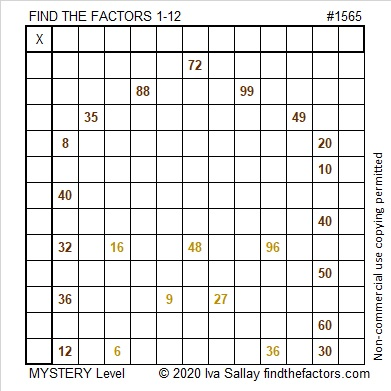### Factors of 1565:

• 1565 is a composite number.
• Prime factorization: 1565 = 5 × 313.
• 1565 has no exponents greater than 1 in its prime factorization, so √1565 cannot be simplified.
• The exponents in the prime factorization are 1 and 1. Adding one to each exponent and multiplying we get (1 + 1)(1 + 1) = 2 × 2 = 4. Therefore 1565 has exactly 4 factors.
• The factors of 1565 are outlined with their factor pair partners in the graphic below.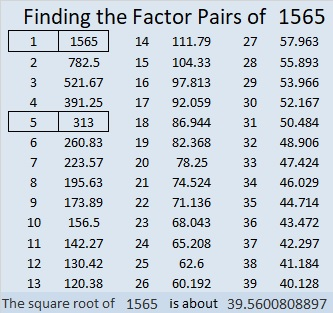### More about the Number 1565:

1565 is the sum of two squares in two different ways:
37² + 14² = 1565, and
38² + 11² = 1565.

125-1560-1565, which is 5 times (25-312-313),
836-1323-1565, calculated from 2(38)(11), 38² – 11², 38² + 11²,
939-1252-1565, which is (3-4-5) times 313, and
1036-1173-1565, calculated from 2(37)(14), 37² – 14², 37² + 14².

# 1563 The Holly Wreath

### Today’s puzzle:

A holly wreath is yet another symbol that connects Christmas with Easter. It symbolizes eternity in its color and shape. It bears white flowers, red berries, and thorns reminding us of purity, blood, and a crown of thorns.

You might find some of the clues in this level 5 puzzle to be like thorns, but don’t give up. Use logic and perseverance and you will be able to find its unique solution.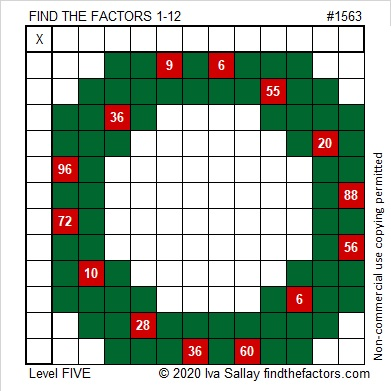Here’s the same puzzle without all the added color: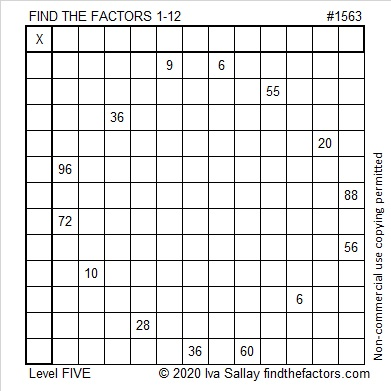### Factors of 1563:

• 1563 is a composite number.
• Prime factorization: 1563 = 3 × 521.
• 1563 has no exponents greater than 1 in its prime factorization, so √1563 cannot be simplified.
• The exponents in the prime factorization are 1 and 1. Adding one to each exponent and multiplying we get (1 + 1)(1 + 1) = 2 × 2 = 4. Therefore 1563 has exactly 4 factors.
• The factors of 1563 are outlined with their factor pair partners in the graphic below.### Another Fact about the Number 1563:

1563 is the hypotenuse of a Pythagorean triple:
837-1320-1563, which is 3 times (279-440-521).

# 1562 Evergreen Tree

### Today’s Puzzle:

An evergreen tree doesn’t drop its leaves in the fall or look dead in the winter. As it reminds us of everlasting life, it makes a lovely symbol of Christmas.Write the numbers from 1 to 10 in both the first column and the top row so that those numbers and the given clues function like a multiplication table. Here is the same puzzle that won’t use as much ink to print:### Factors of 1562:

• 1562 is a composite number.
• Prime factorization: 1562 = 2 × 11 × 71.
• 1562 has no exponents greater than 1 in its prime factorization, so √1562 cannot be simplified.
• The exponents in the prime factorization are 1, 1, and 1. Adding one to each exponent and multiplying we get (1 + 1)(1 + 1)(1 + 1) = 2 × 2 × 2 = 8. Therefore 1562 has exactly 8 factors.
• The factors of 1562 are outlined with their factor pair partners in the graphic below.### Another Fact about the Number 1562:

2(5⁴ + 5³ + 5² + 5¹ + 5⁰) = 1562

# 1558 The Reason for the Season

### Today’s Puzzle:

What is the reason we have the Christmas season? The answer to that question actually begins with a cross…Write the numbers from 1 to 12 in both the first column and the top row so that the given clues are the products of those numbers.

### Factors of 1558:

• 1558 is a composite number.
• Prime factorization: 1558 = 2 × 19 × 41.
• 1558 has no exponents greater than 1 in its prime factorization, so √1558 cannot be simplified.
• The exponents in the prime factorization are 1, 1, and 1. Adding one to each exponent and multiplying we get (1 + 1)(1 + 1)(1 + 1) = 2 × 2 × 2 = 8. Therefore 1558 has exactly 8 factors.
• The factors of 1558 are outlined with their factor pair partners in the graphic below.### Another Fact about the Number 1558:

1558 is the hypotenuse of a Pythagorean triple:
342-1520-1558, which is 38 times (9-40-41).

# 1452 Poinsettia Plant Mystery

### Today’s Puzzle:

Merry Christmas, Everybody!

The poinsettia plant has a reputation for being poisonous, but it has never been a part of a whodunnit, and it never will. Poinsettias actually aren’t poisonous.

Multiplication tables might also have a reputation for being deadly, but they aren’t either, except maybe this one. Can you use logic to solve this puzzle without it killing you?To solve the puzzle, you will need some multiplication facts that you probably DON’T have memorized. They can be found in the table below. Be careful! The more often a clue appears, the more trouble it can be:Notice that the number 60 appears EIGHT times in that table. Lucky for you, it doesn’t appear even once as a clue in today’s puzzle!

### Factors of 1452:

Now I’d like to factor the puzzle number, 1452. Here are a few facts about that number:

1 + 4 + 5 + 2 = 12, which is divisible by 3, so 1452 is divisible by 3.
1 – 4 + 5 – 2 = 0, which is divisible by 11, so 1452 is divisible by 11.

• 1452 is a composite number.
• Prime factorization: 1452 = 2 × 2 × 3 × 11 × 11, which can be written 1452 = 2² × 3 × 11²
• 1452 has at least one exponent greater than 1 in its prime factorization so √1452 can be simplified. Taking the factor pair from the factor pair table below with the largest square number factor, we get √1452 = (√484)(√3) = 22√3
• The exponents in the prime factorization are 2, 1, and 2. Adding one to each exponent and multiplying we get (2 + 1)(1 + 1)(2 + 1) = 3 × 2 × 3 = 18. Therefore 1452 has exactly 18 factors.
• The factors of 1452 are outlined with their factor pair partners in the graphic below.### 1452 Factor Tree:

To commemorate the season, here’s a factor tree for 1452:### More about the Number 1452:

1452 is the difference of two squares three different ways:
364² – 362² = 1452,
124² – 118² = 1452, and
44² – 22² = 1452.

Have a very happy holiday!

# 1450 A Pair of Factor Trees

On today’s puzzle, there are two small Christmas trees. Will two smaller trees on the puzzle be easier to solve than one big one? You’ll have to try it to know!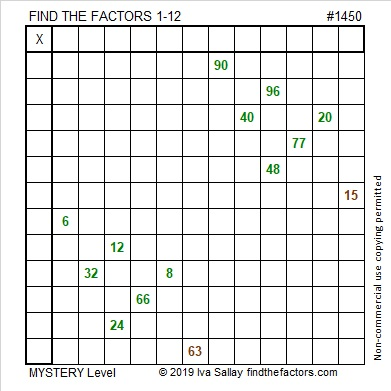Every puzzle has a puzzle number to distinguish it from the others. Here are some facts about this puzzle number, 1450:

• 1450 is a composite number.
• Prime factorization: 1450 = 2 × 5 × 5 × 29, which can be written 1450 = 2 × 5² × 29
• 1450 has at least one exponent greater than 1 in its prime factorization so √1450 can be simplified. Taking the factor pair from the factor pair table below with the largest square number factor, we get √1450 = (√25)(√58) = 5√58
• The exponents in the prime factorization are 1, 2, and 1. Adding one to each exponent and multiplying we get (1 + 1)(2 + 1)(1 + 1) = 2 × 3 × 2 = 12. Therefore 1450 has exactly 12 factors.
• The factors of 1450 are outlined with their factor pair partners in the graphic below.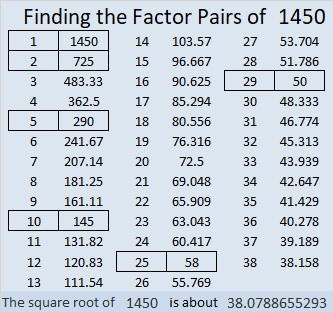In case you are looking for factor trees for 1450, here are two different ones: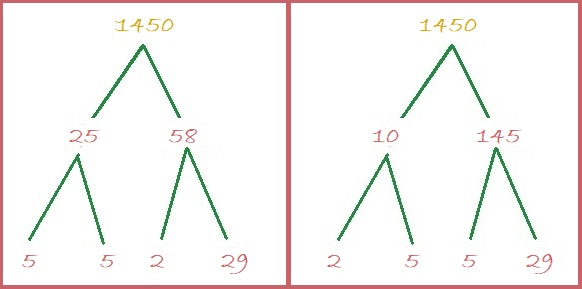1450 is the hypotenuse of SEVEN Pythagorean triples:
170-1440-1450 which is 10 times (17-144-145)
240-1430-1450 which is 10 times (24-143-145)
406-1392-1450 which is (7-24-25) times 58
666-1288-1450 which is 2 times (333-644-725)
728-1254-1450 which is 2 times (364-627-725)
870-1160-1450 which is (3-4-5) times 290
1000-1050-1450 which is (20-21-29) times 50